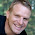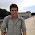## Monday, August 20, 2018

### The And & If Statements

Using And & If Statements to find the median of a pdf

Question:   The table below has data on number of days a stock fund rises in a 10-day period for 242 10-day periods?  Use the And & If statements to find the median of the number of winning days over a 10-day period.

 VDE Up-Day Frequency  for 10 day period X=k Freq K P(X=k) CDF(k) 0 0 0.000 0.000 1 0 0.000 0.000 2 3 0.012 0.012 3 24 0.099 0.112 4 54 0.223 0.335 5 48 0.198 0.533 6 55 0.227 0.760 7 26 0.107 0.868 8 25 0.103 0.971 9 7 0.029 1.000 10 0 0.000 1.000 242 1.000

Comment:  I routinely look at statistics like this for many stocks and I need to modify my spreadsheets so this calculation is updated automatically when I add new data.

Code:   The data (x=0 to x=k)  is in cells range a3:d10.  The CDF in column D is the input.  I place code in column E.

 CDF(k) Col E. 0.000 =IF(D3>=0.5,1,0) 0.000 =IF(AND(D3<0.5,D4>=0.5),1,0) 0.012 =IF(AND(D4<0.5,D5>=0.5),1,0) 0.112 =IF(AND(D5<0.5,D6>=0.5),1,0) 0.335 =IF(AND(D6<0.5,D7>=0.5),1,0) 0.533 =IF(AND(D7<0.5,D8>=0.5),1,0) 0.760 =IF(AND(D8<0.5,D9>=0.5),1,0) 0.868 =IF(AND(D9<0.5,D10>=0.5),1,0) 0.971 =IF(AND(D10<0.5,D11>=0.5),1,0) 1.000 =IF(AND(D11<0.5,D12>=0.5),1,0) 1.000 =IF(AND(D12<0.5,D13>=0.5),1,0)

My final spreadsheet looks like this:

 VDE Up-Day Frequency for 10 day period X=k Freq K P(X=k) CDF(k) Col E 0 0 0.000 0.000 0 5 1 0 0.000 0.000 0 2 3 0.012 0.012 0 3 24 0.099 0.112 0 4 54 0.223 0.335 0 5 48 0.198 0.533 1 6 55 0.227 0.760 0 7 26 0.107 0.868 0 8 25 0.103 0.971 0 9 7 0.029 1.000 0 10 0 0.000 1.000 0

The median occurs for the value k where CDF of K-1 is 0 and CDF of K is 1.     The median is 5 winning days over the sample of 242 10-day periods for this fund.

I tried to extract the value of the median using Lookup function.  It bombed.   However, since there is only one value of column E that has a value of 1 the SUMPRODUCT of col e and col a (the values of k) gives us the median.   This is in cell F3 above.

Authors Notes:

Go to the following post to learn how to use the frequency function in Excel.

1.2.